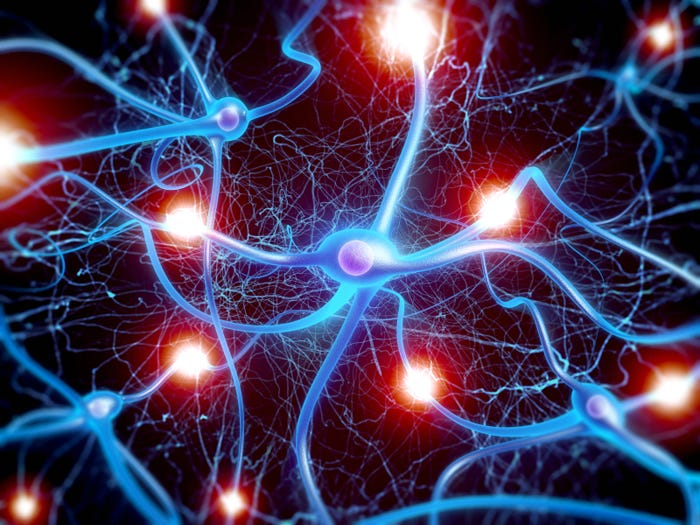# Firing up the neurons: All about Activation Functions

Original article was published on Deep Learning on Medium# Firing up the neurons: All about Activation Functions

Everything you need to know about the activation functions in Deep learning!

“Our intelligence is what makes us human, and AI is an extension of that quality.”
Yann LeCun

While studying deep learning, it is important to understand the underlying concepts of neural networks and their components. It will be easier to code the model after understanding these concepts. Even if you have some knowledge of deep learning and have trained a few models, this article will be helpful. In my previous article, while describing deep neural networks, I mentioned “activation function” quite a few times but I didn’t explain the concept of activation functions in detail. So today we are going to discuss that! If you have a dilemma, which activation function should I use after this layer? What are the pros and cons of that one? Is it necessary to use an activation function? What is the logic behind using any ‘x’ activation function here?

I’ll try to give answers to all such questions below. Still, if you have any other doubt related to activation function post them below in the comment section.

# What is an Activation function?

Briefly describing, an activation function is a mathematical function that helps a neural network to learn complex patterns in the data.
The title of this article, “Firing up the neurons” came up from an analogy between the neurons in the human brain and the neurons in a neural network. When comparing with a neuron-based model that is in our brains, the activation function is at the end deciding what is to be fired to the next neuron. That is exactly what an activation function does in an ANN as well. It takes in the output signal from the previous cell and converts it into some form that can be taken as input to the next cell. The term “activation” is used because it kind-of activates the neuron, just like when you accidentally touch a hot pan and the neurons get fired up and send the signal to your brain, and thus you can react quickly.
Although I don’t find this analogy that much pleasing because even the neuroscientists can’t firmly tell about the working of neurons in a human brain. But many deep learning books and courses give this analogy, so I thought that maybe I share it here too.

All you have to know that activation functions are different types of Mathematical functions that are mainly used to learn complex patterns in data. Though different types of activations have further different uses which we will discuss in a minute.

# Need for Activation Functions

Activation functions learn complex patterns by adding a non-linearity. Linear activation functions are rarely used (only in regression problems) but mainly we use non-linear activation functions in deep learning.
Have a look in the image below:

The first graph is linear classification, but this data distribution is too good to be true in deep learning. Remember when I told you about the structured and unstructured data. This graph seems to have structured data distributed.
The second graph shows a non-linear classification but it overfits! (Will talk about it later).
But the third one the inseparable one can’t be classified accurately with linear activation functions. So we need activation functions to add non-linearity to our data. It also has a mathematical proof that concludes the same but I thought this graph comparison will be easier to understand when starting off.

# Properties of Activation Functions

Some of the general properties of activation functions are:

1. Differentiable: We have to differentiate all the neurons during the backpropagation to optimize the loss function. That’s why all the activation functions are differentiable.
2. Computational Expense: Activation functions are applied after every layer and need to be calculated millions of times in deep networks. Hence, they should be computationally inexpensive to calculate.
3. Vanishing Gradient problem: Some activation functions gives rise to the vanishing gradient problem. We will discuss this later in detail when we will discuss other problems in deep learning models. For intuitive understanding, you can think that as we go containing the neural network, instead of converging the loss to its optimal value, the gradients shift towards zero. This causes the gradients to be such small that it takes much time to converge into the global optimum or it even can’t reach that point.
4. Monotonicity: Most of the activation functions are monotonic in nature i.e it is either entirely non-decreasing or entirely non-increasing.
Note: Activation functions are monotonic not their derivatives.

If you are not familiar with calculus, the above properties can be difficult to understand.
But……

The properties of activation functions do not matter much. You only need to know how to use the activation functions and what they do.

Moving on, let’s talk about different types of activation functions:

# Types of Activation Functions

## 1. Rectified linear unit (ReLU):

The rectified linear unit or simply ReLU is one of the widely used activation functions. It simply outputs the input as it is, if it is a positive number and if it is a negative number, it outputs zero.
Mathematically: